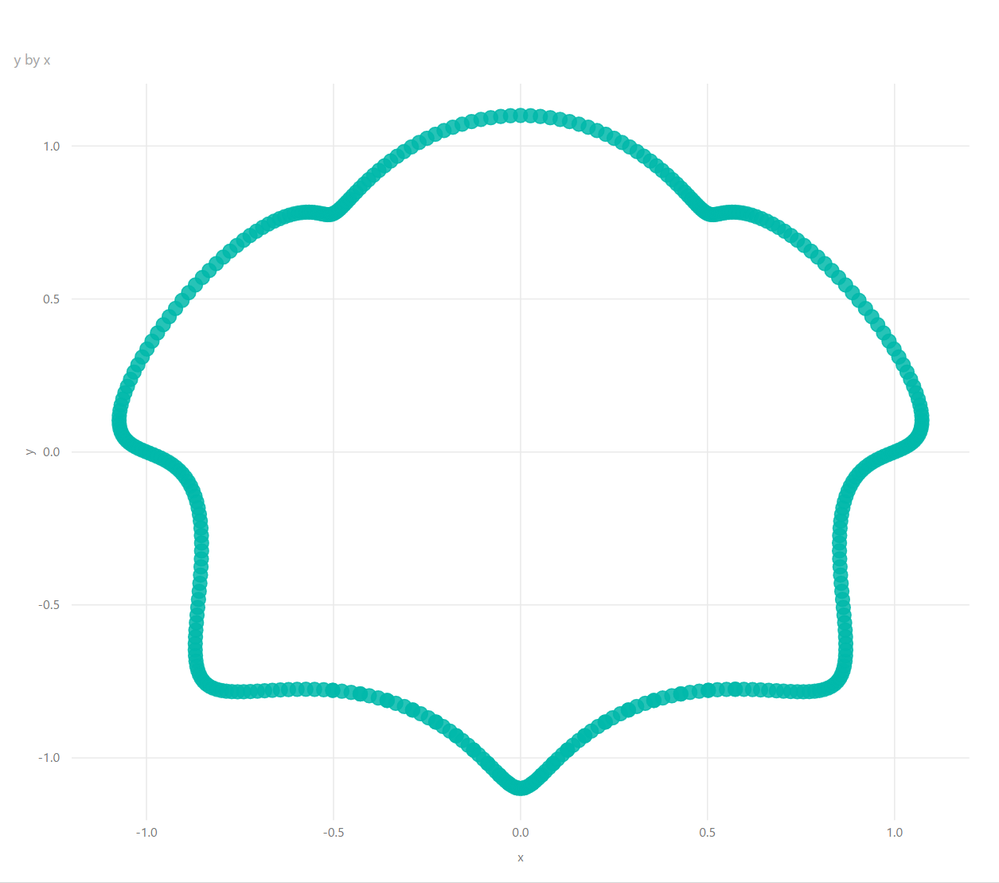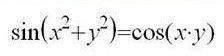cancel
Showing results for
Did you mean:## Fun with Graphing in Power BI - Part SQRT(4)

Introduction

Ah yes, stupid math humor. It's like the i talks to pi joke. You know, the one where i says to pi "Be rational!" and pi says to i, "Get real!". No? Oh well. You're probably better off that way.

Anyway, so what have we learned from Fun with Graphing in Power BI - Part 1 thus far? I mean other than the fact that mathematicians and biologists apparently hate everyone? Well, hopefully we have learned a thing or two about some of the cool graphing that we can do in Power BI and perhaps a thing or two about polar coordinates, radians and the like. So, let's continue our exploration of graphing in Power BI. I'm going to present a few more interesting plots using the same basic technique as last time and then I'll show off a new trick for generating plots for really nasty equations. Nasty!

Same Old Same Old But Cool

First up is what I call the "kidney" plot. In Power BI Desktop, create a New Table from the Modeling tab and plug in the following formula:

`Kidneys = GENERATESERIES(0, 10, .001)`

Rename the Value column to "t" and then create two new columns with the following formulas:

```x = 2*COS([t])+SIN(2*[t])*COS(60*[t])

y = SIN(2*[t])*SIN(60*[t])```

OK, now create a Scatter chart visual and put y in the Y Axis and x in the X Axis. Remember, you may have to switch it to a Line chart visual, drag x to the Axis. Then switch back to a Scatter chart visual and drag y to the Y Axis and then x to the X Axis again. Why? No idea...but it works. Proving once again that you don't have to have any conceivable notion of how things like microwaves and non-dairy creamer work in order to use them.

What you should end up with is something like this:Kinda 3-D looking, right? The cool part of this graph is that you can watch it "grow". So, click on your table and edit the formula and change the 10 to 1 and then 2, 3, 4 and so on and you can watch it start plotting the right "kidney" then the left "kidney". Neat.

The next one I call the "muffin". Same drill. Create a new table with the following formula:

`Muffin = GENERATESERIES(-10, 10, .05)`

Rename Value to "t" and then the following two columns:

```x = SIN([t])+1/10*SIN(6*[t])
y = COS([t])+1/10*COS(7*[t])```

Your Scatter chart should end up looking like this:Finally, let's get biological! This next plot ends up looking like some sort of leaf. I'm not sure what type of leaf it is, but my buddies out in Colorado really seem to like this one. They told me to call it the 420 plot, whatever that means...

Use this formula for your new table:

`420 = GENERATESERIES(-3.141592654, 3.141592654, 0.001)`

Rename Value to t again. And then these three columns:

```r = (1 + 0.9 * COS(8*[t])) * (1 + 0.1 * COS(24*[t])) * (0.9 + 0.05 * COS(200*[t])) * (1 + SIN([t]))

x = [r] * COS([t])

y = [r] * SIN([t])```

Plot x and y in a Scatter chart and you should get an interesting looking leaf shape:Let's Step it Up a Notch

OK, enough with the same old technique. You get the idea. Let's move on to some really nasty equations. You see, with their infinite capacity for utter cruelty and hatred toward mankind, mathematicians will often write formulas for Cartesian plots like the following:Now, the problem is that trying to expand and simplify trigonometric functions to get these types of formulas into terms of x and y is like crazy PhD level hard. Luckily, I actually know someone with a PhD in engineering. Unfortunately, it is my older brother, Dan. And while it almost killed me to do it, I reached out to him for help. No, seriously, it really did almost kill me. You see, whenever I even remotely consider asking my older brother for help, I instinctively get a running start and smash my head into a wall. This technique generally works quite well because by the time I wake up, I have forgotten that I was going to embarrass myself and ask my older brother for help.

Unfortunately, in this particular case either I got a bit too much of a running start or I encountered an extra hard wall because I ended up on the sidewalk bleeding profusely from a gaping head wound. In my delirium, I apparently texted my brother the following:

"Dan, I was going to ask you about how to plot the formula sin(x^2+y^2)=cos(x*y) when I slammed my head into this wall and now I'm bleeding really badly. I need help. I don't want to die."

So Dan, being an older brother, responded back immediately with the answer to the graphing problem and told me to stop being dramatic. Typical. Luckily for me though, a passerby happened to call 911 and I met a very nice paramedic who bandaged up my head and was extremely sympathetic. Turns out, he also has an older brother, so he could definitely relate. He even showed me his own head scars! Really nice guy.

So, since I did not "technically" actually ask my brother for help with plotting the formula but rather to help me from dying...which he ignored, and I am not the least bit bitter about...I don't really feel embarrassed to share with you the technique Dan shared with me while I was bleeding profusely about the head the neck...on the sidewalk...dying...I'm just saying.

So, what you want to do is, you guessed it, create a new table with the following formula:

`Graph = GENERATESERIES(-10,10,.001)`

Rename the Value column to x. Now, and here's the tricky part, create ANOTHER new table with the formula:

`yTable = GENERATESERIES(-10,10,.1)`

Rename the Value column to y.

Now, back in your Graph table, create the following column:

```y = VAR yTable1 = ALL(yTable[y])
VAR yTable2 = ADDCOLUMNS(yTable1,"z",SIN(POWER([x],2)+POWER([y],2)) - COS([x]*[y]))
VAR MINz = MINX(FILTER(yTable2,[z]>=0),[z])
RETURN MAXX(FILTER(yTable2,[z]=MINz),[y])```

So, what's going on here? Well, what you do is simplify the equation thus:

SIN(POWER([x],2)+POWER([y],2)) = COS([x]*[y]))

becomes:

SIN(POWER([x],2)+POWER([y],2)) - COS([x]*[y])) = 0

You then "invent" the variable "z" that you substitute for the zero. Now, you pick a value for x and you solve for each value of "y". When z is zero, that is the answer for the equation for the value of y at that value of x. Rinse and repeat for another value of x. So, with the two tables for x and y our new column does all of this heavy lifting for us so to speak. Nifty.

Now you can plot x and y from your Graph table on a Scatter chart and get something like the following:Even better, we can simply choose another formula and create another column in our Graph table like this one:

```y2 = VAR yTable1 = ALL(yTable[y])
VAR yTable2 = ADDCOLUMNS(yTable1,"z",COS(SIN([x]*[y])+COS([x])) - SIN(SIN([x])+COS([y])))
VAR MINz = MINX(FILTER(yTable2,[z]>=0),[z])
RETURN MAXX(FILTER(yTable2,[z]=MINz),[y])```

Now, if we plot x and y2 from our Graph table on another Scatter chart we get some "scribbles", which are really cool:Conclusion

Alright, so I will leave you with one final plot that, due to my fear of reprisals from the #MeToo movement, I will only provide you with the equations and not the final plot. This last plot, along with proving that there is apparently a mathematical equation for literally just about everything, also demonstrates that while mathematicians are obviously innately cruel and hateful beings, that they are also perhaps a bit sexually frustrated to boot.

New table:

`Mystery = GENERATESERIES(.001,1,.001)`

Rename Value column to y

And these columns:

`x = 3*[y] * LOG([y]) - 1/36*EXP(-1*POWER(36*[y]-36/EXP(1),4))Color = IF([x]<=-.48,1,0)`

You know what to do. Enjoy!

Posts in this series: 1, 2, 3, 4, 5